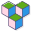CppBuzz# Java program to find largest among three numbers

``````public class Main {

public static void main(String[] args) {
int x=100;
int y=120;
int z=100;

//1. approach
if(x>y && x>z) {
System.out.println("x is the greatest number");
}
else if(y>z) {
System.out.println("y is the greatest number");
}
else {
System.out.println("z is the greatest number");
}

//2. approach
if(x>=y) {
if(x>=z) {
System.out.println("x is the greatest number");
}
else {
System.out.println("z is the greatest number");
}
}
else {
if(y>=z) {
System.out.println("y is the greatest number");
}
else {
System.out.println("z is the greatest number");
}
}
}
}``````